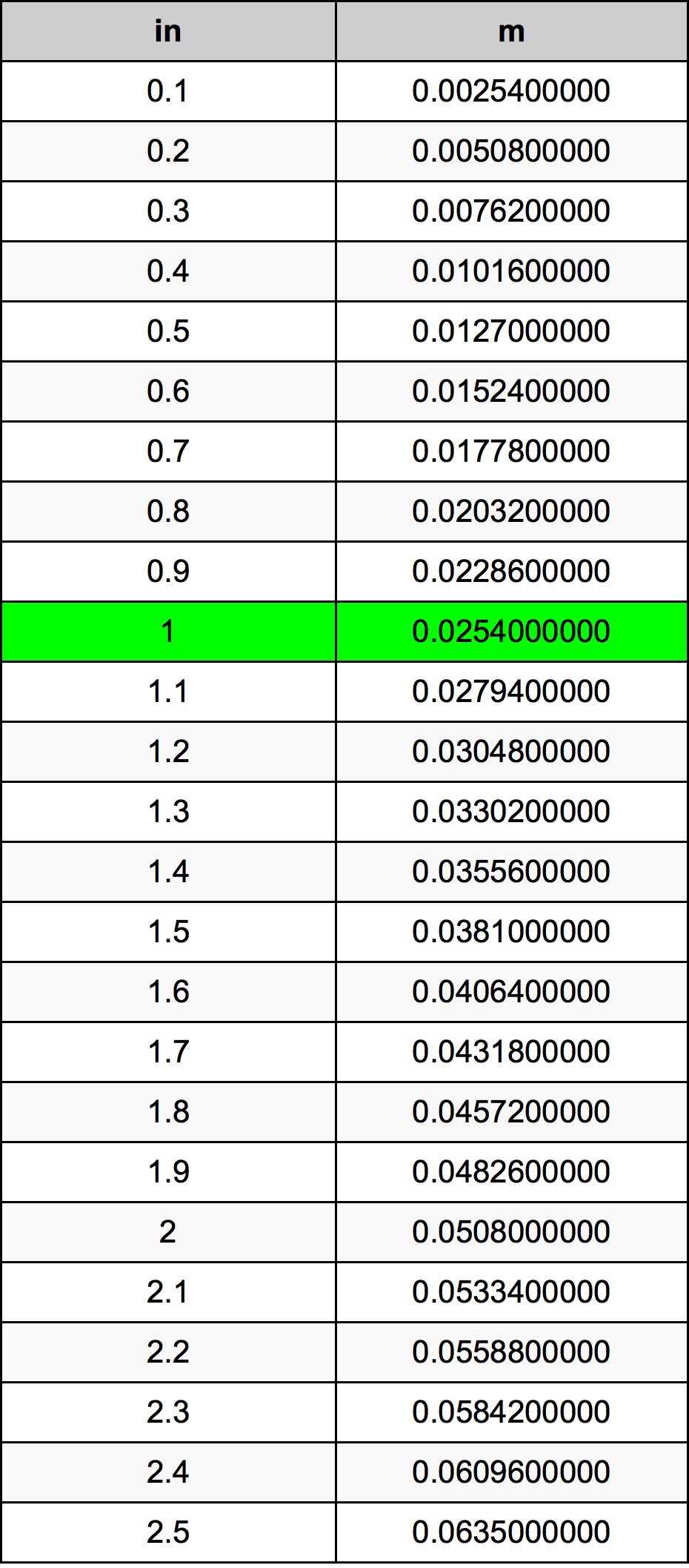Inches To Meters

# 1 in to m1 Inch to Meters

in
=
m

## How to convert 1 inch to meters?

 1 in * 0.0254 m = 0.0254 m 1 in
A common question is How many inch in 1 meter? And the answer is 39.3700787402 in in 1 m. Likewise the question how many meter in 1 inch has the answer of 0.0254 m in 1 in.

## How much are 1 inches in meters?

1 inches equal 0.0254 meters (1in = 0.0254m). Converting 1 in to m is easy. Simply use our calculator above, or apply the formula to change the length 1 in to m.

## Convert 1 in to common lengths

UnitLengths
Nanometer25400000.0 nm
Micrometer25400.0 µm
Millimeter25.4 mm
Centimeter2.54 cm
Inch1.0 in
Foot0.0833333333 ft
Yard0.0277777778 yd
Meter0.0254 m
Kilometer2.54e-05 km
Mile1.57828e-05 mi
Nautical mile1.37149e-05 nmi

## What is 1 inches in m?

To convert 1 in to m multiply the length in inches by 0.0254. The 1 in in m formula is [m] = 1 * 0.0254. Thus, for 1 inches in meter we get 0.0254 m.

## 1 Inch Conversion Table## Alternative spelling

1 Inches to Meters, 1 Inches in Meters, 1 in to Meters, 1 in in Meters, 1 Inch to m, 1 Inch in m, 1 Inch to Meters, 1 Inch in Meters, 1 Inches to m, 1 Inches in m, 1 Inch to Meter, 1 Inch in Meter, 1 Inches to Meter, 1 Inches in Meter